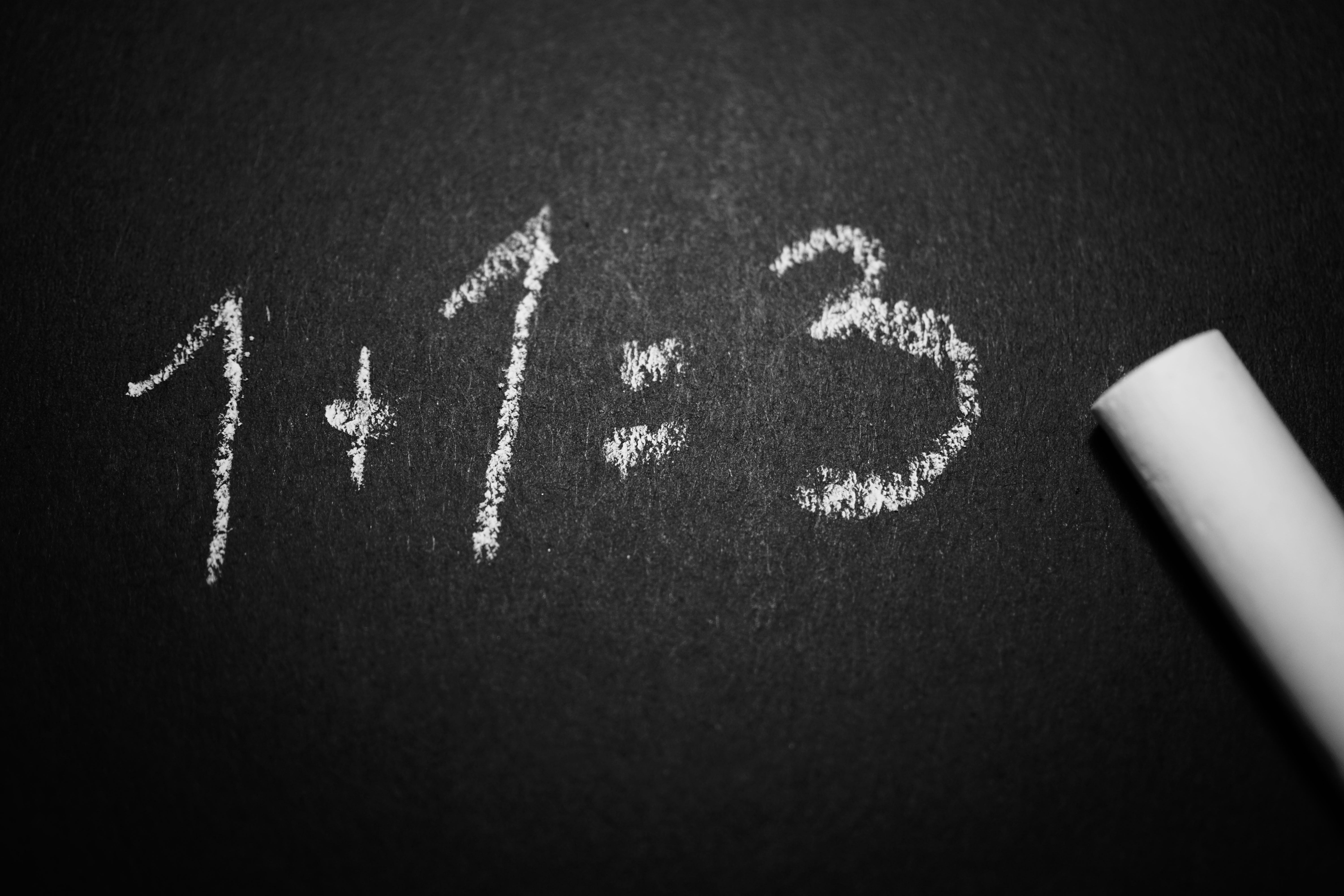# What is the hardest math class?

What is the Hardest Math Class?

# What is the Hardest Math Class?

As a college tips and tricks blogger, I often get asked about the hardest math course that students may encounter. In this article, we’ll explore different opinions and factors that contribute to determining the hardest math class.

## FAQ List

1. ### What is the hardest math course in high school?

The hardest math course in high school typically depends on the individual student and the school’s curriculum. However, in most cases, you’ll find that AP Calculus BC or IB Math HL is the most difficult math course your school offers. Note that AP Calculus BC covers the material in AP Calculus AB but also continues the curriculum, addressing more challenging and advanced concepts.

2. ### What makes a math course difficult?

Several factors contribute to the difficulty of a math course, including:

• The abstract nature of mathematical concepts: Some students may struggle to understand concepts that they cannot see or touch.
• The pace of the course: Faster courses may leave some students behind and overwhelmed.
• The level of rigor: Certain mathematical concepts may require a greater level of critical thinking and analysis, which may be challenging for some students.
• The quality of teaching: Teachers with different teaching styles, experience and knowledge may influence the level of difficulty of the math course.
3. ### What are some tips to succeed in a difficult math class?

Some tips to help students succeed in a difficult math class include:

• Attend all your lectures and take detailed notes.
• Engage in active learning like participating in class discussions or forming study groups.
• Get help when you need it. If you don’t understand a concept or question, consult your teacher or teaching assistant, classmates, or contact online math tutoring sites.
• Practice regularly and allocate enough time for your homework and assignments so you can absorb the concepts without rushing.
4. ### What is the hardest college math course?

Just like in high school, the hardest math course in college may vary depending on the students’ perspectives, professors and programs. However, higher-level mathematics courses like abstract algebra, real analysis, and topology often pose some of the greatest challenges for students earning their bachelor’s degrees in mathematics.

5. ### What do students in the toughest math classes learn?

Most of the difficult math classes focus on abstract and complex concepts that require higher-level analytical skills. Examples of difficult mathematics topics students may learn in complex math courses include multivariable calculus, number theory, differential equations, abstract algebra and topology.

## Different Opinions on the Hardest Math Class

There are several opinions on what the hardest math class is. Some students may find Geometry challenging because of the abstract concepts, proofs and theorems. Other students may enjoy Geometry but struggle with Algebra, especially when it involves manipulating multiple variables and functions.On the other hand, some students may find Calculus the hardest math class they’ve taken. Calculus is divided into two courses – Calculus I and Calculus II. Calculus I deals with subjects, such as limits, derivatives, integrals, and techniques of differentiation, and Calculus II covers topics like sequences, series, polar coordinates and complex numbers.However, some students may argue that Linear Algebra or Probability is the most difficult math course. Linear Algebra is all about matrices, vectors, and systems of linear equations, while Probability deals with the study of random events, statistics and likelihoods.There are also higher and more difficult math courses like Multivariable Calculus, Differential Equations, Number Theory, and Applied Mathematics that students can take depending on their interests and program requirements.Ultimately, the difficulty of a math course comes down to personal opinions, work ethic and how well the subject matter matches a student’s interest and skills. Thus, a course like Multivariable Calculus may be the hardest math class for some students but an easy one for others who love visual mathematics, calculus and 3D concepts.